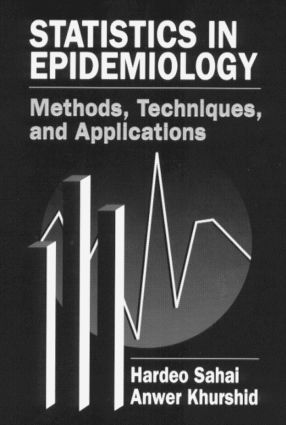Statistics in Epidemiology

Methods, Techniques and Applications, 1st Edition

CRC Press

352 pages

Purchasing Options:\$ = USD
Hardback: 9780849394447
pub: 1995-12-21
SAVE ~\$48.00
Currently out of stock
\$240.00
\$192.00
x

FREE Standard Shipping!

Description

Epidemiologic studies provide research strategies for investigating public health and scientific questions relating to the factors that cause and prevent ailments in human populations. Statistics in Epidemiology: Methods, Techniques and Applications presents a comprehensive review of the wide range of principles, methods and techniques underlying prospective, retrospective and cross-sectional approaches to epidemiologic studies. Written for epidemiologists and other researchers without extensive backgrounds in statistics, this new book provides a clear and concise description of the statistical tools used in epidemiology. Emphasis is given to the application of these statistical tools, and examples are provided to illustrate direct methods for applying common statistical techniques in order to obtain solutions to problems.

Statistics in Epidemiology: Methods, Techniques and Applications goes beyond the elementary material found in basic epidemiology and biostatistics books and provides a detailed account of techniques:

Reviews

"This book is an excellent review of fundamental and advanced statistical methods for the analysis of epidemiologic data. The authors cover the derivations of important epidemiologic indices with substantial mathematical detail and applications to various study designs. …Another strength is the care that the authors have taken to give appropriate software choices for each technique cited. …the mathematical thoroughness with which the measures are covered earns this text a high recommendation as a reference text for the practicing quantitative epidemiologist's desktop."

-Journal of the American Statistical Association

"The book focuses explicitly on epidemiological studies…Other positive features are the absence .of any content devoted to derivations in spite of the extensive and explicit presentation of all the relevant equations…and the inclusion of a numerical example for every one of the computational procedures that is shown…Exceptional literature reference lists follow each of the 11 chapters…"

-Techonometrics

"This volume is designed as a core handbook for a course in statistics applied to epidemiology…The presentation is accurate and proceeds slowly from crude to stratified estimation, comparing at every step alternative procedures for the calculation of point estimates and confidence intervals…This volume will be welcomed by many clinical epidemiologists for its clear structure and extensive listing of the methods so often cited in the literature."

-International Statistical Review

"…clearly achieves wide coverage of the important features of statistical epidemiology."

-Peter Armitage, Professor Emeritus of Applied Statistics, Oxford University, U.K.

Preface

Introduction

Types of Epidemiologic Study Designs

Prospective vs. Retrospective Study Designs. Cross-Sectional vs. Longitudinal Studies. A Note on Terminology. The Measurement of Effect. Design-Based vs. Model-Based Inference.

Prospective (Cohort) Studies

Measures of Risk: Risk Difference. Risk Ratio. Odds Ratio. A Comparison of Taylor Series and Test-Based Methods. Risk Ratio vs. Odds Ratio.

Retrospective (Case-Control) Studies

Measures of Risk: Risk Ratio. Odds Ratio.

Cross-Sectional Studies

Measures of Prevalence. Point and Interval Estimation.

Stratified Analysis

Confounding and Interaction. Adjustment for Confounding in a 2 x 2 Table. Measures of Risk. Measures of Prevalence.

Matching

Matched-Pair Analysis. Matched-Set Analysis Involving Multiple Controls Per Case. Matched-Set Analysis Using Varying Number of Controls Per Case. Efficacy of Matching. Matched Follow-Up Studies.

Multivariate Models

Linear Models. Binary Logistic Regression. Proportional Hazards Regression. Poisson Regression.

Prospective (Cohort) Studies with Person-Time Data

Measures of Risk: Incidence Rate Difference. Incidence Rate Ratio.

Stratified Analysis with Person-Time Data

Measures of Risk. Other Summary Effect Measures.

Attributable Risk

Percent Attributable Risk. Population Attributable Risk. Confidence Intervals. Adjustment for Confounding.

Appendices

Pearson Chi-Square vs. Mantel-Haenszel Chi-Square. Variance of a Function of a Random Variable. The Odds Ratio. More Confidence Intervals for the Risk Ratio. Maximum Likelihood Estimation. Variance of the Conditional Maximum Likelihood Estimator of the Odds Ratio and the Log Odds Ratio. Variance of the Maximum Likelihood Estimator of the Common Odds Ratio in a Stratified Analysis. Some Important Probability Distributions. Randomized and Mid-p Tests and Mid-p Confidence Intervals. Mantel-Haenszel Chi-Square Statistic for Stratified 2 x 2 Tables. Analysis of a 2 x l Table. Maximum Likelihood Estimation for the Logistic Regression Model. Logistic Regression Model for a Case-Control Design. Odds Ratio as an Approximation to a Risk Ratio.

Exercises

References

Related Bibliography

Indexes

Author Index. Subject Index. Data Index.